##### The Japanese Maple specialist
Direct order Contact Help / Services Newsletter# Shelf to displaySearch

Japanese MaplesBonsai soil and fertiliserAcer seeds and more Young maples and bonsaiHostasWind bells and chimesSaxifragesVarieties introduced into Europe

Packing and shipping charges

# Stewartia monadelpha 0.5 litre pot 30 cm

Shimeshara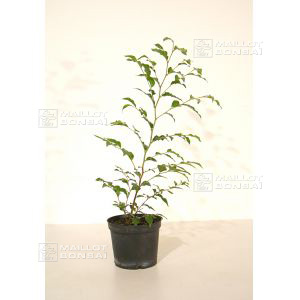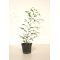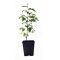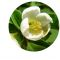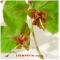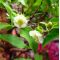ref. : 10230

12,00

Available quantity : 77Order

###### Description

Descended from a 2/3 year-old seedling.

Delivered in a plastic 0.5 litre pot.

Height 30 cm.

Stewartia monadelpha is an outstanding tree for year-round interest. At the beginning of summer white cupped flowers bloom, while in winter the leaves drop to reveal a red-orange bark. In spring pink foliage emerges, in summer it turns green and in autumn it is red-orange. The tree prefers cool soils in summer and a mid-shade position. This beautiful species is often used in bonsai.

#monadelpha 4 #stewartia 3.9 #summer 3.6 #bonsai 3.6 #litre 3.5 #orange 2.6 #maples 2.6 #young 2.5 #year 2.4 #tree 2.4

Formule
(( ROUND((CHAR_LENGTH(b.article_nom)-CHAR_LENGTH(REPLACE(b.article_nom, 'monadelpha', '')))/LENGTH('monadelpha')) + ROUND((CHAR_LENGTH(b.article_description)-CHAR_LENGTH(REPLACE(b.article_description, 'monadelpha', '')))/LENGTH('monadelpha')) ) * 4) + (( ROUND((CHAR_LENGTH(b.article_nom)-CHAR_LENGTH(REPLACE(b.article_nom, 'stewartia', '')))/LENGTH('stewartia')) + ROUND((CHAR_LENGTH(b.article_description)-CHAR_LENGTH(REPLACE(b.article_description, 'stewartia', '')))/LENGTH('stewartia')) ) * 3.9) + (( ROUND((CHAR_LENGTH(b.article_nom)-CHAR_LENGTH(REPLACE(b.article_nom, 'summer', '')))/LENGTH('summer')) + ROUND((CHAR_LENGTH(b.article_description)-CHAR_LENGTH(REPLACE(b.article_description, 'summer', '')))/LENGTH('summer')) ) * 3.6) + (( ROUND((CHAR_LENGTH(b.article_nom)-CHAR_LENGTH(REPLACE(b.article_nom, 'litre', '')))/LENGTH('litre')) + ROUND((CHAR_LENGTH(b.article_description)-CHAR_LENGTH(REPLACE(b.article_description, 'litre', '')))/LENGTH('litre')) ) * 3.5) + (( ROUND((CHAR_LENGTH(b.article_nom)-CHAR_LENGTH(REPLACE(b.article_nom, 'orange', '')))/LENGTH('orange')) + ROUND((CHAR_LENGTH(b.article_description)-CHAR_LENGTH(REPLACE(b.article_description, 'orange', '')))/LENGTH('orange')) ) * 2.6) + (( ROUND((CHAR_LENGTH(b.article_nom)-CHAR_LENGTH(REPLACE(b.article_nom, 'tree', '')))/LENGTH('tree')) + ROUND((CHAR_LENGTH(b.article_description)-CHAR_LENGTH(REPLACE(b.article_description, 'tree', '')))/LENGTH('tree')) ) * 2.4) + (( ROUND((CHAR_LENGTH(b.article_nom)-CHAR_LENGTH(REPLACE(b.article_nom, 'year', '')))/LENGTH('year')) + ROUND((CHAR_LENGTH(b.article_description)-CHAR_LENGTH(REPLACE(b.article_description, 'year', '')))/LENGTH('year')) ) * 2.4) + (( ROUND((CHAR_LENGTH(b.article_nom)-CHAR_LENGTH(REPLACE(b.article_nom, 'outstanding', '')))/LENGTH('outstanding')) + ROUND((CHAR_LENGTH(b.article_description)-CHAR_LENGTH(REPLACE(b.article_description, 'outstanding', '')))/LENGTH('outstanding')) ) * 2.1) + (( ROUND((CHAR_LENGTH(b.article_nom)-CHAR_LENGTH(REPLACE(b.article_nom, 'beautiful', '')))/LENGTH('beautiful')) + ROUND((CHAR_LENGTH(b.article_description)-CHAR_LENGTH(REPLACE(b.article_description, 'beautiful', '')))/LENGTH('beautiful')) ) * 1.9) + (( ROUND((CHAR_LENGTH(b.article_nom)-CHAR_LENGTH(REPLACE(b.article_nom, 'delivered', '')))/LENGTH('delivered')) + ROUND((CHAR_LENGTH(b.article_description)-CHAR_LENGTH(REPLACE(b.article_description, 'delivered', '')))/LENGTH('delivered')) ) * 1.9)

## Secure payment## Delivery

Our logistic partners :